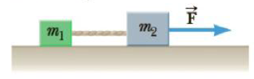Chapter 4, Problem 55P

Chapter
Section
Textbook Problem

A block of mass m1 = 16.0 kg is on a frictionless table to the left of a second block of mass m2 = 24.0 kg, attached by a horizontal string (Fig. P4.55). If a horizontal force of 1.20 × 102 N is exerted on the block m2 in the positive x-direction, (a) use the system approach to find the acceleration of the two blocks. (b) What is the tension in the string connecting the blocks?Figure P4.55

(a)

To determine
The acceleration of the two blocks.

Explanation

Given Info: Mass ( m1 ) is 16.00 kg and Mass ( m2 ) is 24.00 kg. The horizontal force applied (F) has a magnitude of 1.20×102N .

The free body diagram is given below.

The two block can be considered as a single block of mass (m1+m2) . Formula for the acceleration is,

a=Fm1+m2

Substitute 1.20×102N for F, 16

(b)

To determine
The tension in the string.

Still sussing out bartleby?

Check out a sample textbook solution.

See a sample solution

The Solution to Your Study Problems

Bartleby provides explanations to thousands of textbook problems written by our experts, many with advanced degrees!

Get Started

Find more solutions based on key concepts